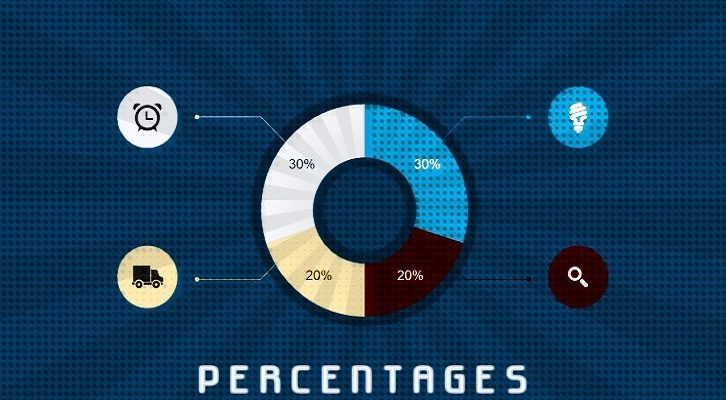# Percentages Solutions

Have given below solution to the final question on percentages .

6. A, B, C and D share a loot. A gets a% of the total. B gets b% of the remaining (after A has taken his share). C gets c% of the remaining and D gets the rest. D gets a% less than what A gets. B and C get equal amounts. b = 2a.
i) What percentage of what A got did C get?
ii) If the total amount is equal to Rs. 1000, what is the difference between what A got and what D got?

Let total amount = T.

A would have got T * a. After A takes his share, there would be T (1-a) left
B would have got T (1-a) * b
C would have got T (1-a)(1-b) * c
D would have got T (1-a)(1-b)(1-c)

D gets a % less than what A gets, or, T *a (1-a) = T (1-a)(1-b)(1-c), or

a = (1-b)(1-c) ——– Eqn 1

B and C get the same amounts, or (1-a) * b = (1-a)*(1-b)*c, or b = (1-b) c, or, b = c – cb, or

b = c/c+1 ———— Eqn 2
b = 2a —————–Eqn 3

Substituting, eqn 2 in and 3 in eqn 1, we get

c/2(c+1) = (1-c)/(c+1)
Or, c = 2 -2c, or c = 2/3

b = c/(c+1) = 2/5, a = 1/5
a gets 20% of the loot, b gets 40% of the remaining 80%, or 32% of the loot. C gets 66.6% of 48%, or 32% of the overall loot. And d gets the final 16% of the overall amount (20% lesser than A’s share)

Now, to the questions

i) What percentage of what A got did C get?

A got 20% of overall, C got 32% of overall. Or, C got 160% of A

ii) If the total amount is equal to Rs. 1000, what is the difference between what A got and what D got?
A would have got Rs. 200, D Rs. 160. A would have got Rs. 40 more than D.